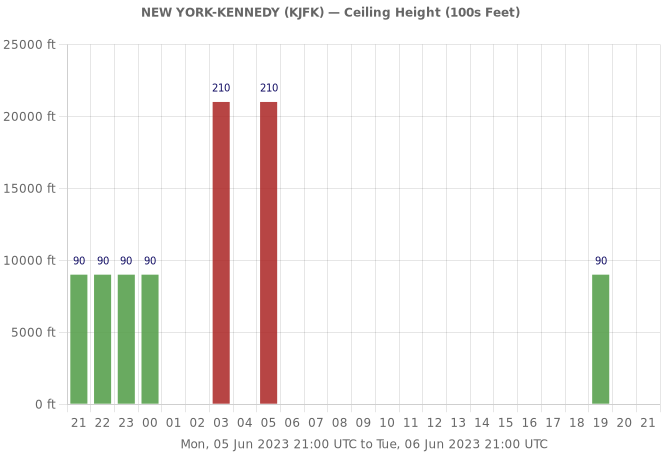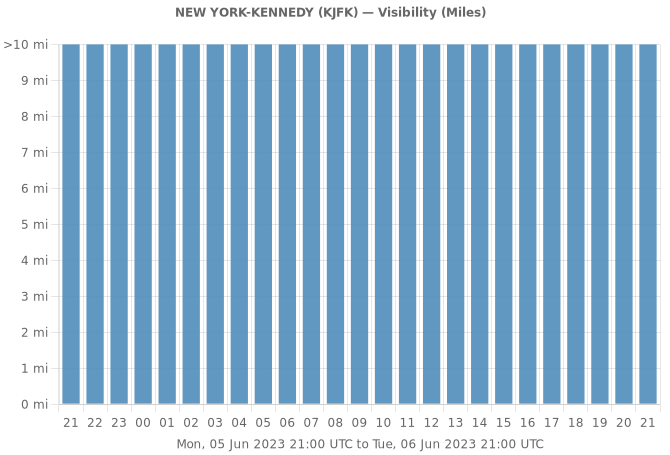Graph data points have three colors. Data points in RED are the maximum value for the graph. GREEN data points are the minimum value. All other data points in between, and data points that are all the same (ie. maximum = minimum), are displayed in BLUE.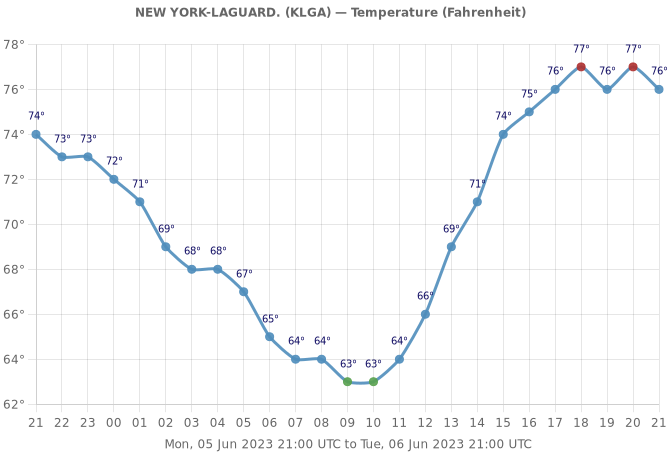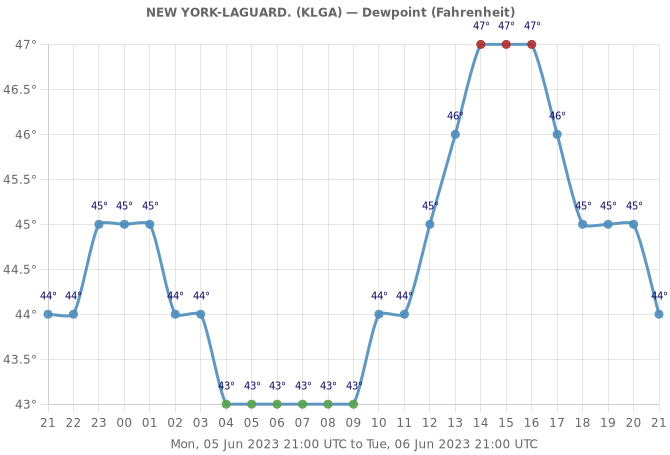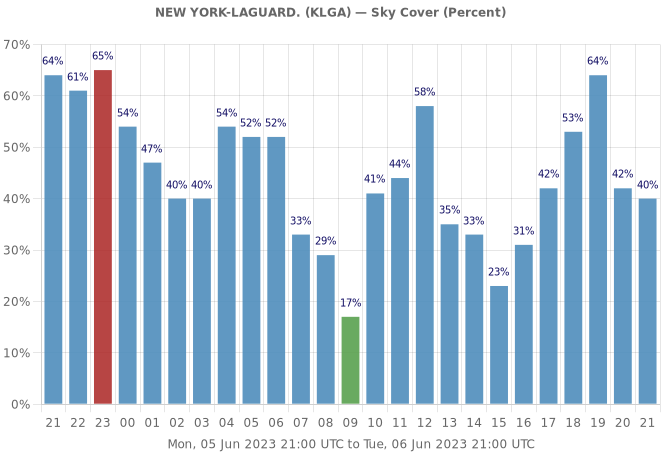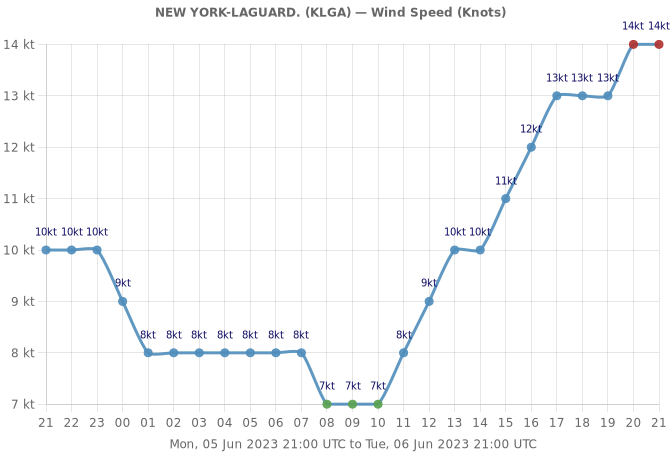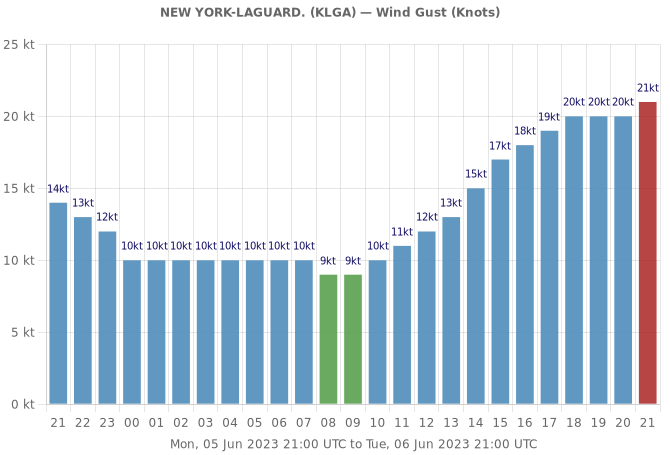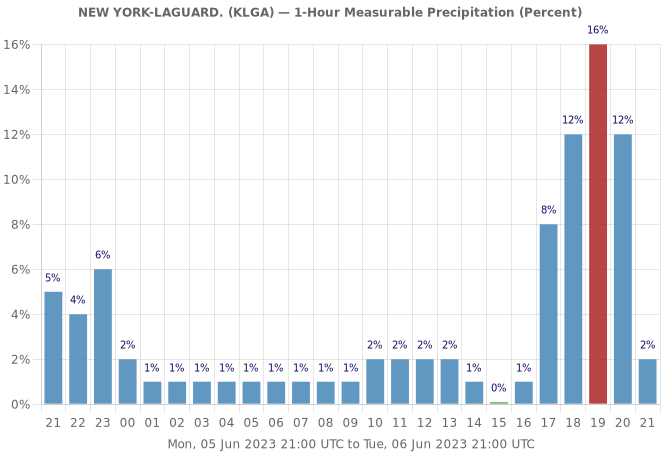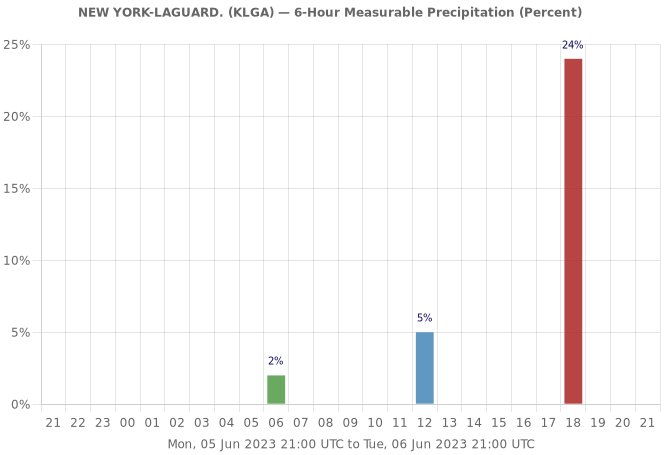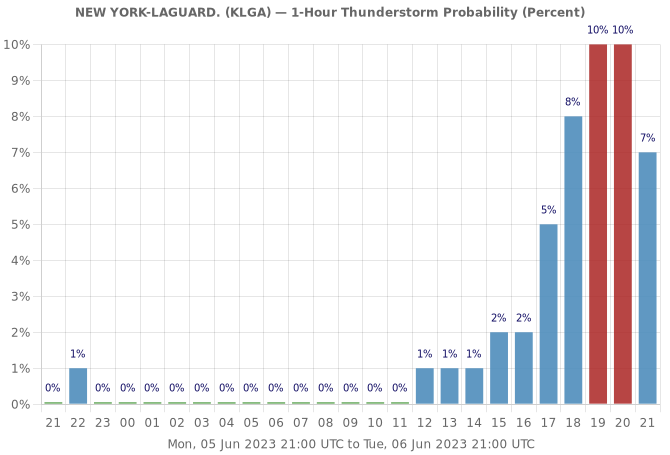The Conditional Probability of Freezing Rain (Percent) data field for NEW YORK-LAGUARD. (KLGA) is zero for the entire period.

The Conditional Probability of Snow (Percent) data field for NEW YORK-LAGUARD. (KLGA) is zero for the entire period.

The Conditional Probability of Sleet (Percent) data field for NEW YORK-LAGUARD. (KLGA) is zero for the entire period.

The 1-Hour Snow Amount (1/10 inches) data field for NEW YORK-LAGUARD. (KLGA) is zero for the entire period.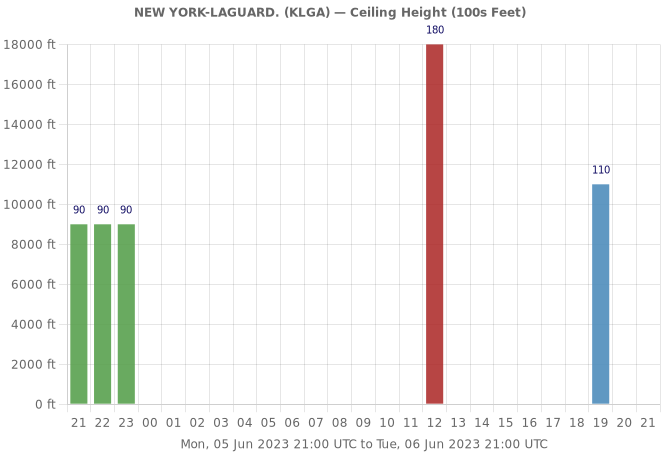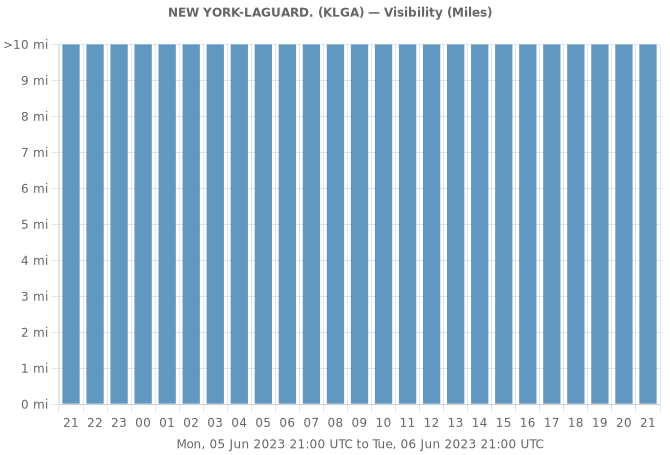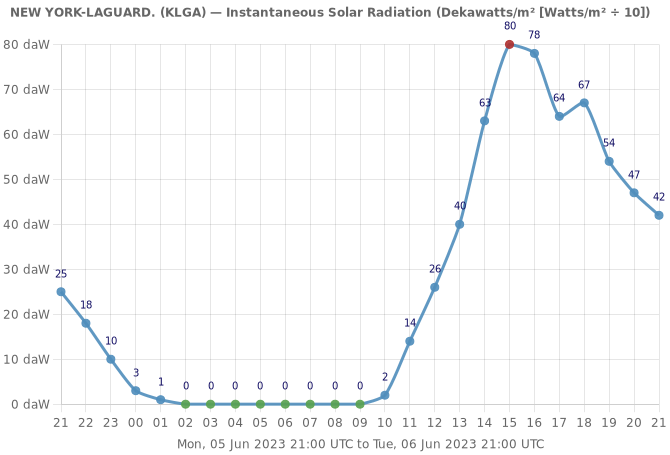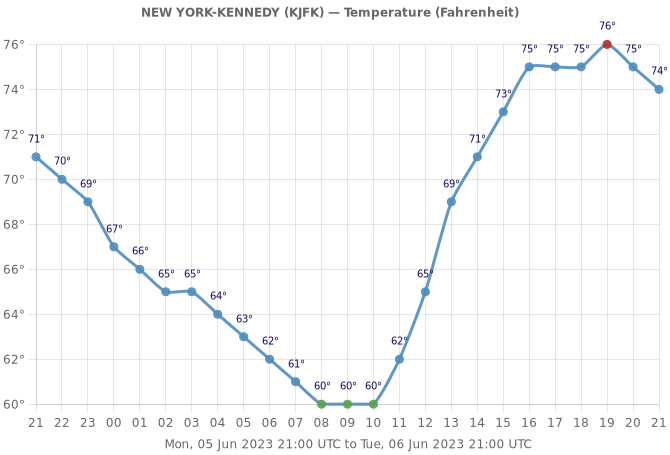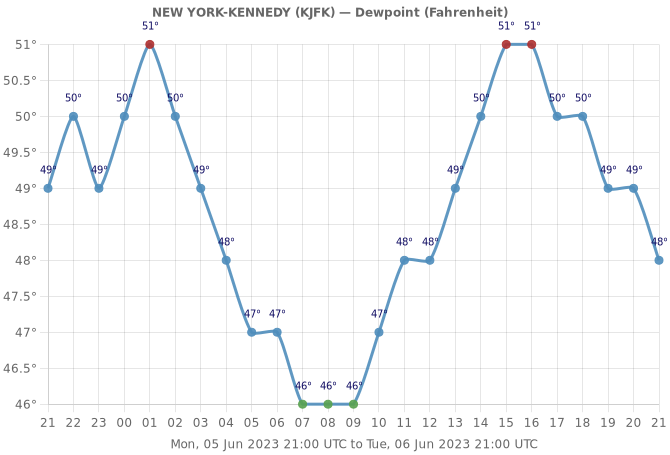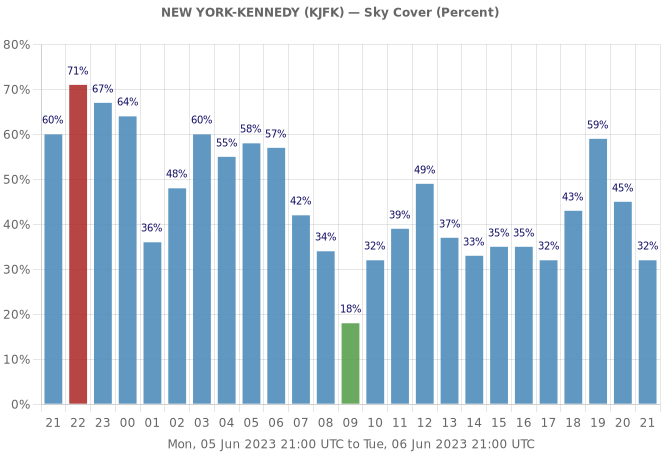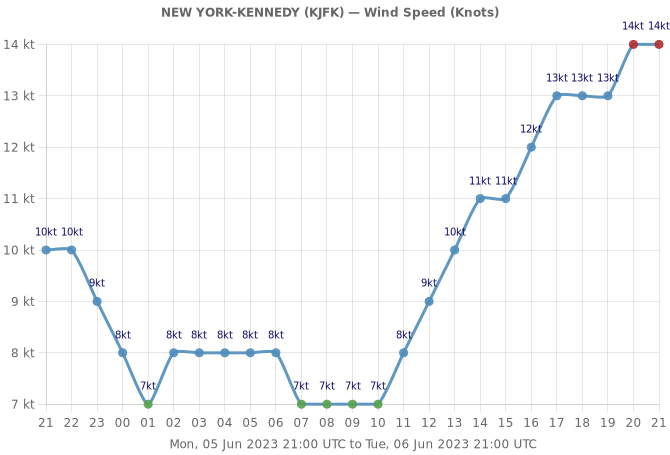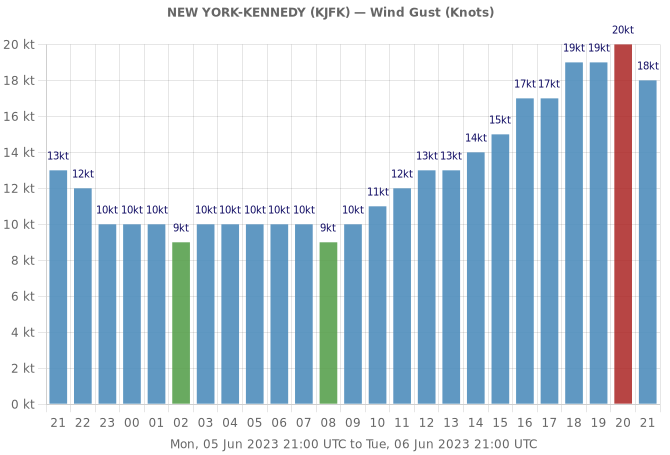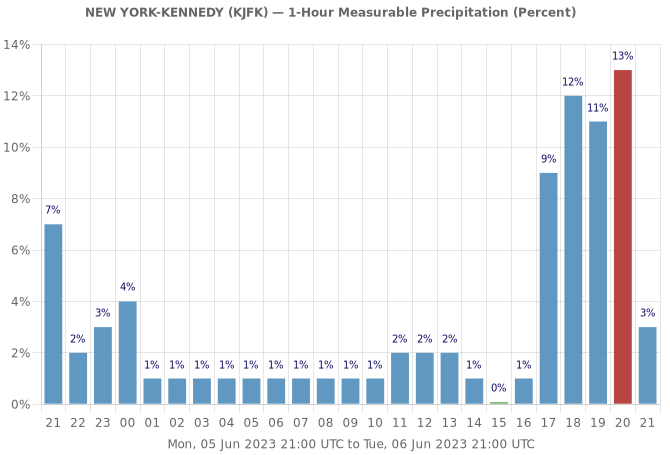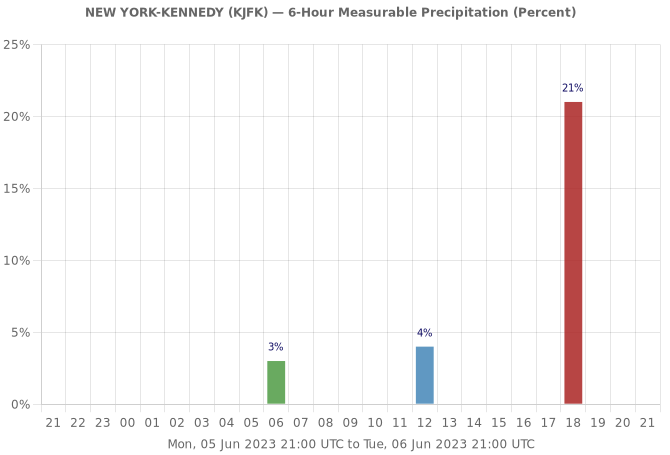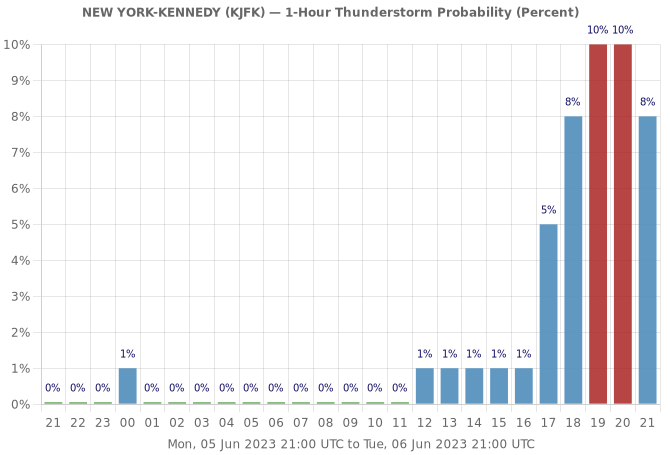The Conditional Probability of Freezing Rain (Percent) data field for NEW YORK-KENNEDY (KJFK) is zero for the entire period.

The Conditional Probability of Snow (Percent) data field for NEW YORK-KENNEDY (KJFK) is zero for the entire period.

The Conditional Probability of Sleet (Percent) data field for NEW YORK-KENNEDY (KJFK) is zero for the entire period.

The 1-Hour Snow Amount (1/10 inches) data field for NEW YORK-KENNEDY (KJFK) is zero for the entire period.